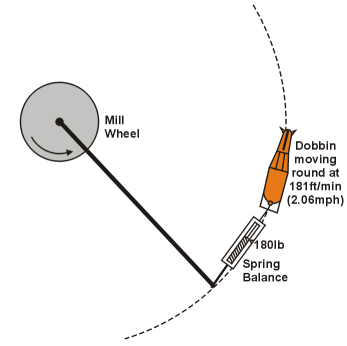Operational Theory

 ****JavaScript based drop down DHTML menu generated by NavStudio. (OpenCube Inc. - http://www.opencube.com)****"More steam, more steam", The Captain cried, "For we are sorely pressed"

And from the bank, the Engineer replied, "The 'orse is doin' 'is best!"

In the days before the steam and internal combustion engines, horses supplied a lot of the energy required to provide motive power.

When James Watt developed the steam engine he wanted to know how the work output of his engine could be measured in a way that non technical people could understand. He had the idea that if he could measure the work output of a horse in a set amount of time he could then use this unit to show how much work his engine could do in the same time, or how many horses it could replace.

In those early days the units of measurement used were the pound for mass, and foot for distance. If a pound in mass was lifted vertically against the force of gravity through 1 foot the amount of work done was 1 foot pound (force). If you lifted 2 pounds through 2 feet against gravity the work done was 4 foot poundsf (the distance × force). The difference between work and power is how fast the work is done; the faster you lift the mass over a given distance, the more power is developed

There are various stories concerning how James Watt (and others) arrived at their calculations. One story recounts that James Watt used a horse at a coal mine which was used to winch the coal (and flood water) from the mine. He found that the horse could do about 16500 foot poundsf of work in one minute (maybe lift 165 pounds through 100 feet). However, due to the losses in friction on the winding gear and acknowledging that the mine horse was not in the peak of condition he multiplied his result by two, giving a result of 33000 foot poundsf in one minute or 550 foot poundsf in one second. This unit he called the horsepower (hp).In other stories he multiplied his result of 22000 by 1.5, and others recount that he determined a horse exerted a force of 180 lbf whist walking in a circle turning a mill wheel. He calculated that the horse walked round in the circle at 181 feet/min. 180 × 181= 32580, which he rounded up to 33000 foot poundsf /min.  (32580/33000 = 0.987)

Unfortunately his calculations were not very accurate. An average horse in good condition can develop 2-3 hp for a short period of time but about 0.7hp continuously over a working day. A reasonably fit person can develop about 0.3 hp whist riding a bike and a really fit athlete can peak at 2.5 horsepower briefly and develop over 1 hp for short periods of time.

Before the introduction of railways a series of canals were dug across the UK to transport goods and raw materials by barge. These barges were towed along by large draft horses (hence the towpath running along the side of a canal). A barge moving at a low speed in still water and not creating a bow wave does not take much power to move. From calculation a 15 metre barge moving at about 3mph (4.43ft/s or 1.35m/s or about 2.6 knots) requires a pull of about 75lbf and  takes about 0.6hp. (well within the capabilities of a horse). So if we wanted to replace the horse with an engine in the barge driving a propeller, what would the required output of the engine? The answer is NOT 0.6 hp

This is because a propeller is only about 50% efficient, so, in reality an engine output of 1.2 hp would be required. If the speed was to double to 6mph the power would have to increase 8 fold to 9.6 hp. (this is because power is proportional to speed3)

A large modern tanker moving at 15knots which is about 25.26 ft/s or 7.7m/s requires a pull (or push) of about 220,000lbf  or 100,000kgf. In other words, according to Watt, about 10,000 horses. To generate this it would require an engine with a shaft output of about double.  i.e 20,000hp.

RELATIONSHIP BETWEEN HP AND KW

The SI units of work and power are the Joule (J) and the Watt (W) or multiples thereof (kJ. MJ, kW,MW etc) The foot poundf as a unit of work is a little confusing. The same unit (the pound) is a unit of mass; when it is used it as part of the unit of work it is acted upon by gravity and becomes a force (hence the subscript f)

Force = mass × acceleration and the unit of force is the Newton (N)

The SI unit of mass is the kg. and the acceleration due to gravity is 9.81m/s2 so the unit of force exerted by 1kg when acted upon by gravity is 1 × 9.81 = 9.81kgm/s2  = 9.81 N

The SI unit of distance is the metre (m)

Work = force (N) × distance (m).

The SI unit for work is the Nm or the Joule (J). 1Nm = 1J

The SI unit of power is the watt = 1 joule of work in one second.

So, if 1kg is lifted vertically against the force of gravity through 1metre the work done is 1 × 9.81 × 1 = 9.81Nm or Joules

and if this work is done in 1second then the power developed is 9.81 Watts.

To convert horsepower to watts:

1 foot = 0.3048metres and there are 2.2 pounds in a Kg

550 foot poundsf /sec × 0.3048 = 167.64 metre poundsf /sec

167.64 ÷ 2.2 = 76.2 metre Kgf/sec

76.2 × 9.81 = 747.522 Nm (Joules)/sec = 747.522 watts

In other words to convert horsepower to watts multiply by 747.522 (750 approx)

Or to convert horsepower to Kilowatts multiply by 0.747522 (0.75 approx)

To convert Kw to horsepower divide by 0.747522 (0.75 approx)